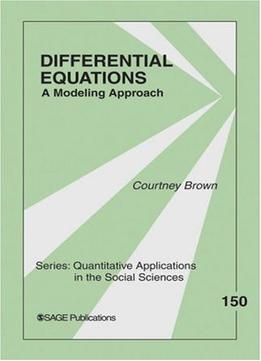# Differential Equations: A Modeling Approach by Courtney Brown / 2007 / English / PDF

Differential Equations: A Modeling Approach introduces differential equations and differential equation modeling to students and researchers in the social sciences. The text explains the mathematics and theory of differential equations. Graphical methods of analysis are emphasized over formal proofs, making the text even more accessible for newcomers to the subject matter. This volume introduces the subject of ordinary differential equations ― as well as systems of such equations ― to the social science audience. Social science examples are used extensively, and readers are guided through the most elementary models to much more advanced specifications. Emphasis is placed on easily applied and broadly applicable numerical methods for solving differential equations, thereby avoiding complicated mathematical “tricks” that often do not even work with more interesting nonlinear models. Also, graphical methods of analysis are introduced that allow social scientists to rapidly access the power of sophisticated model specifications. This volume also describes in clear language how to evaluate the stability of a system of differential equations (linear or nonlinear) by using the system’s eigenvalues. The mixture of nonlinearity with dynamical systems is a virtual trademark for this author’s approach to modeling, and this theme comes through clearly throughout this volume. This volume’s clarity of exposition encourages social science students of mathematical modeling to begin working with differential equation models that address complex and sophisticated social theories.

views: 614